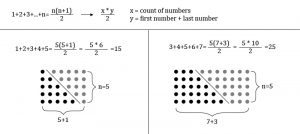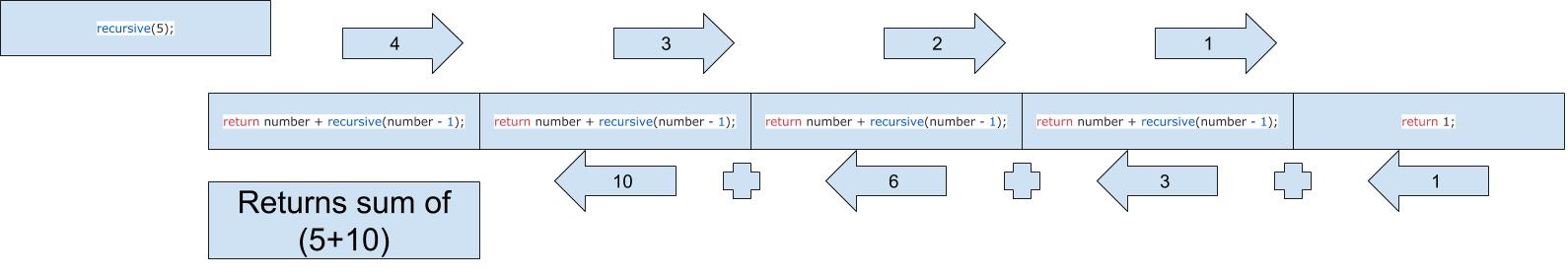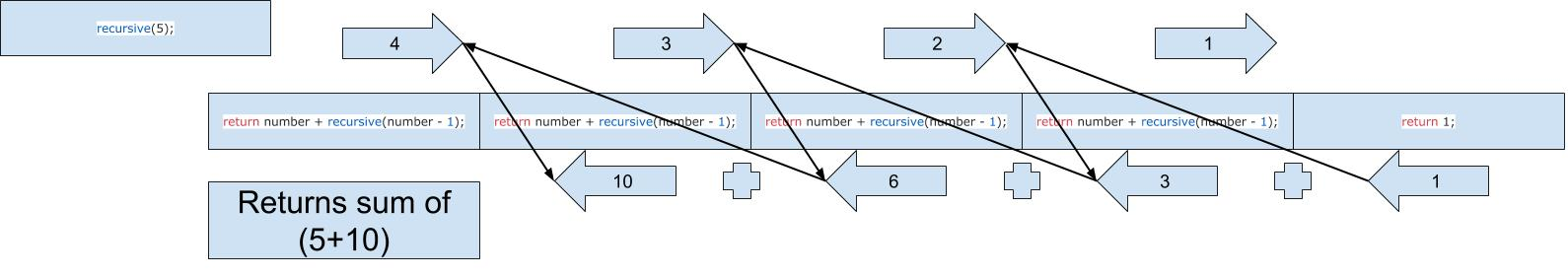# CS50 Week 2: Shorts Part 2

These next 3 shorts, binary search, recursion, and merge sort were very frustrating at first. Don’t get discouraged. Take lots of breaks, and use the repl.it website so you can write code and see the results quickly. My goal was to write each of these without looking at any reference material, and it was very rewarding to complete merge sort by reasoning everything out in my head. It took around 2 weeks for me until I got to that point. There were times when I wanted to throw my computer out the window!

## Binary Search

Unlike linear search (also called sequential search), binary search allows us to remove about half the array on each pass in it’s search for our target number. We only need to satisfy one requirement, which is the array has to be sorted.

This is a good time to get comfortable with understanding bounds, and a quick refresher on the way int data types are handled when we divide them.

Let’s look at our array:

```02,04,06,08,10,12,14,16,18 // array values
00,01,02,03,04,05,06,07,08 // array positions
#S,xx,xx,xx,#M,xx,xx,xx,#E // variable positions

target value: 2```

We start off with the current state. The while loop is going to update the state of our array with the knowledge that it is sorted. The strategy of finding the target value n is this:

• While start is equal to or less than end
• check if our mid value is equal to our target and if so break out and print that it is found. If it is not true…
• If the value is less than the mid value, update the state so we focus on everything to the left of mid
• otherwise (if greater than mid) update the state so we focus on everything to the right of mid

So our while loop runs so long as start is <= mid. What does that mean?

It means we want to stay within our bounds. We don’t want to touch array[-1] or array, so this condition protects us from that.

Checking the mid value is where we’ll find our target eventually if it’s in the array. Notice the break out part because once we find the target we want to stop looping.

If we don’t find our target value we have to update the state of our program. Since we’re cutting the array in about half some variables are going to have to change. This way our while loop can keep doing it’s thing, using our new variables.

Let’s look at some pseudo code:

```02,04,06,08,10,12,14,16,18 // array values
00,01,02,03,04,05,06,07,08 // array positions
#S,xx,xx,xx,#M,xx,xx,xx,#E // variable positions

start = 0
end = 8
mid = (0+8)/2 which is 4

target is 2

// making first pass through the while loop
checking if mid(4) is equal to target(2)...
NO!

checking if target(2) is less than mid(4)
YES!
UPDATING VARIABLE POSITIONS
start is unchanged!
end = mid(4) - 1 which is 3
mid = start(0) + end(3) / 2

// 3 divided by 2 is 1.5
// the result is truncated because we are dealing with ints!
// so now mid is position 1

Starting while loop again with new state:
02,04,06,08 // array values
00,01,02,03 // array positions
#S,#M,xx,#E // variable positions```

So we cut the array in half by updating 2 variables. We are always going to update the middle variable. See how middle isn’t actually in the middle when we update the state of our array? It all works out in the end anyways, just something to keep in mind.

Let’s do this again but with a target value to the right of our first mid value.

```02,04,06,08,10,12,14,16,18 // array values
00,01,02,03,04,05,06,07,08 // array positions
#S,xx,xx,xx,#M,xx,xx,xx,#E // variable positions

start = 0
end = 8
mid = (0+8)/2 which is 4

target is 18

// making first pass through the while loop
checking if mid(4) is equal to target(18)...
NO!

checking if target(2) is less than mid(4)
NO!

target(2) MUST be greater so execute the else block:

UPDATING VARIABLE POSITIONS
start is mid + 1 which is 5
end is unchanged!
mid = start(5) + end(8) / 2
// 5+8 = 13
// 13 / 2 = 6.5
// the result is truncated because we are dealing with ints!
// so now mid is position 6

Starting while loop again with new state:
12,14,16,18 // array values
05,06,07,08 // array positions
#S,#M,xx,#E // variable positions```

```02 // array values
00 // array positions
#S // variable positions (they are all the same!)
#E
#M

start = 0
end = 0
mid = (0+0)/2 which is 0

target is 1

// making first pass through the while loop
checking if array[mid(0)] is equal to target(1)...
NO!

Value is less than 2 so updating state...
start is unchanged! (start still equal to 0)
end is mid - 1 which is -1
mid is -1 - 0 which is - 1

going back to while loop start...
checking while loop condition...
start is greater than end!
out of bounds!```

So in either situation. Going too far to the left makes the end variable a negative number, leaving start at zero. This makes start(0) greater than end(-1), and so we’re out of bounds. On the other side, if end is the last array position, let’s say position 8 and we put start beyond that at (9), start(9) is again larger than end(8) and we’re out of bounds.

TIP

I made the mistake at first of leaving out array[variable] and just using variable in my if statements. This meant I was comparing variable instead of array[variable]. One checks the actual value in the array and the other is just the position number. Line 26 is a perfect example. I kept using just mid and didn’t realize why things weren’t working!

Get comfortable with this because the next two are not easier!

## Recursion

This technique allows us to call a function within itself. In order to do this and not get stuck in an infinite loop, we have to have a base case. Something has to be checked each time we call (or re-call) our function. That something needs to satisfy our base case’s condition at some point, and finally we’ll return our function (and all the recursive calls).

Let’s see this in action with a simple function:

So the function recursive() takes a number as it’s only argument, and will recursively calculate what’s know as the nth triangle. This visual helps us understand the reason it’s called that:Source: https://goo.gl/H5oTqR

How does this happen using recursion? Line 16 is where we make the call to our function from within, but instead of number we are sending number-1. Each call eventually leads to our number being equal to 1, and then we’re hitting our base case on line 13. Think of this as a guard making sure our function doesn’t create an infinite loop.

Sometimes visuals help us understand abstract ideas like recursion. I’ve never liked the stack frame images popping off the stack, so this is an image i’ve created that works for me. Here is a visual of our recursive function calls:And here is another image with lines connecting to where things get added up coming back from return 1 (follow the lines ex: 1+2=3 then 3+3=6 etc):Keep in mind no work is actually returned until we hit the base case. The calls are like a rubber band being pulled until finally we hit return 1 and all the other functions get returned going back to the first time it was called (the arrows pointing left is the final snap of everything returning back).

The bottom arrow with 1 is saying “ok here I am returning one” which the second to last call has been waiting for. It’s holding number which at that time is 2. You can see i’ve drawn a little line at the tip of the arrow holding 1 at the far bottom right. That little line means it’s completing the calculation 1+2 which is our arrow with the number 3. Now follow the small lines that represent the math calculation giving us our numbers below that eventually lead back to the original function giving us our original number 5 plus everything added up from the recursive calls.

I strongly recommend creating your own version of a recursive function!

See if you can create a recursive function that works. Create some printf statements and try to predict the outcome of the recursion. Be patient!

## Merge Sort

This algorithm was discovered by John Von Neumann in 1945. Using recursion, elements in an array are split until the array is of size 1. Then we re-combine the sorted sets over and over until we have a single sorted array. Merge sort has a runtime of O(n log n) which means every time the number of elements doubles, only 1 more step is added to processing time.

I have created 2 versions for you to study. One is a basic version that only covers the sorting, while the other version sorts and merges. I’ve included lots comments that explain my thought process through each step.

basic:

Full merge sort:

## GDB

The final short explains how to debug a program using gdb. I’ve written a little playground program we can use to test out some interesting tricks that will help us understand the state of our programs at precise moments in time. This ability to freeze time and look at the state of our variables within a certain scope is very helpful. Once you get into this habit of using a debugger, it will make printf statements seem silly (although they are a lot more convenient when you are just starting out and I personally still use them more than I should). Here is the code:

I’ve created this file in the CS50 IDE with the name test.c so to run this with gdb i’ll type in the terminal:

`~/workspace/ \$ gdb test`

Let’s just use gdb to figure out two things:

1. the state of our variables in specific points in our code. Maybe at a certain line or maybe a certain iteration within a loop. Maybe inside a function call.
2. The data types of our variables.

Let’s look at our code output for a moment:

```~/workspace/ \$ ./test
hw
party time! Hello | 42 | m
97
98
99```

Line 8 shows an array of chars, but the output is numbers when printed out. Why does this happen?

Since we can’t figure out why, we can examine where first then use the state of the variables to give us a clue.

Line 15 is our printf statement so let’s examine things there. To run the debugger we just type run or just r. Unfortunately doing this will run our program from start to finish just like running it in the terminal. We want to pause somewhere in the program and “look around.”

In gdb this is called a break. Type break or just b followed by the line you’d like to stop at. Let’s break at line 15.

```~/workspace/ \$ gdb test
(gdb) break 15
Breakpoint 1 at 0x42064c: file test.c, line 15.
(gdb) run
Starting program: /home/ubuntu/workspace/test
hw
party time! Hello | 42 | m

Breakpoint 1, main () at test.c:15
15 printf("%i\n", x[i]);
(gdb)```

I’ve put the important lines in bold to help clarify what is going on. We run gdb on our file test.c, we want to break on line 15 and run. The last line shows we’re on line 15 and prints the expression to the terminal. Let’s test 2 things, the entire expression on line 15 and then the variable we are interested in. Also keep in mind we want to know the value and the data type:

```(gdb) whatis printf("%i\n", x[i])
type = int
(gdb) whatis x[i]
type = char
(gdb) print printf("%i\n", x[i])
97
\$1 = 3
(gdb) print x[i]
\$2 = 97 'a'
(gdb)```

So we see the expression gives us a data type int and the variable alone gives us a char. Something is definitely wrong here because we want to print a char. So we test print the entire expression and get 97. When we print the variable we conveniently get both 97 and the letter ‘a.’ Hopefully this was enough of a clue to remind you that chars have corresponding integers and printf has specific formatters %i and %c. So we need to change our expression.

So how do we look at other stuff? What if we want to check the next iteration of our loop?

Try this command once your on line 15 and gdb is running:

```Breakpoint 1, main () at test.c:15
15 printf("%i\n", x[i]);
(gdb) c
Continuing.
97

Breakpoint 1, main () at test.c:15
15 printf("%i\n", x[i]);
(gdb) print x[i]
\$1 = 98 'b'
(gdb)```

Notice how printing x[i] gives us the next item in our array. Using the c command (continue) we are continuing through to the next iteration of the loop. NOTE: the command works this way because our breakpoint is right where we want to be in the loop.

You can also move around using the n or next command. Let’s set a new break point right at the beginning of our program. This is usually done by simply typing b main or break main. We can also print local variables using info locals and see nearby lines of code by typing l (as in list). Now our program starts on line 6. Let’s go through line by line using n:

```~/workspace/ \$ gdb test
(gdb) break main
Breakpoint 1 at 0x4205a9: file test.c, line 6.
(gdb) r
Starting program: /home/ubuntu/workspace/test

Breakpoint 1, main () at test.c:6
6 printf("hw\n");
(gdb) l
1 #include <stdio.h>
2
3 void party_time();
4
5 int main(void){
6 printf("hw\n");
7
8 char x[] = {'a','b','c','d'};
9
10 int length = sizeof x / sizeof x;
(gdb) n
hw
8 char x[] = {'a','b','c','d'};
(gdb) n
10 int length = sizeof x / sizeof x;
(gdb) n
12 party_time();
(gdb) n
party time! Hello | 42 | m
14 for(int i = 0; i < length-1; i++)
(gdb) n
15 printf("%i\n", x[i]);
(gdb) n
97
14 for(int i = 0; i < length-1; i++)
(gdb) n
15 printf("%i\n", x[i]);
(gdb) n
98
14 for(int i = 0; i < length-1; i++)
(gdb) n
15 printf("%i\n", x[i]);
(gdb) n
99
14 for(int i = 0; i < length-1; i++)
(gdb) n
19 return 0;```

Now let’s dive into that function party_time using the s command which means to “step” into a function, changing our local scope. Now info locals will be completely different. I’ll break a line before the function, use to go right on the party_time line and then s to step in it and check info locals again from within:

```~/workspace/ \$ gdb test
(gdb) b 10
Breakpoint 1 at 0x4205bc: file test.c, line 10.
(gdb) r
Starting program: /home/ubuntu/workspace/test
hw

Breakpoint 1, main () at test.c:10
10 int length = sizeof x / sizeof x;
(gdb) info locals
x = "abcd"
length = 32767
(gdb) n
12 party_time();
(gdb) s
party_time () at test.c:23
23 char* x = "Hello";
(gdb) n
24 int y = 42;
(gdb) info locals
x = 0x4276f3 "Hello"
y = 32767
z = -9 '7'
(gdb) n
25 char z = 'm';
(gdb) info locals
x = 0x4276f3 "Hello"
y = 42
z = -9 '7'
(gdb) finish
Run till exit from #0 party_time () at test.c:25
party time! Hello | 42 | m
main () at test.c:14
14 for(int i = 0; i < length-1; i++)
(gdb)```

So let’s go over the play by play

I startup gdb with file test

break on line 10

run

I check the local variables that exist at that moment on line 10 and we find:

x = “abcd”
length = 32767

What is length that funky number? Remember we are on line 10 not past line 10 which means it hasn’t been evaluated, so it’s a garbage value! If we did the next command and checked again it would be the actual value we want. Back to the play by play:

I go to the next line using n

now that i’m on the same line as the function I want to dive into I type s to step inside

I’m teleported to line 23

I use the next command to go to the following line 24

I check the local variables and get:

x = 0x4276f3 “Hello”
y = 32767
z = -9 ‘\367’

y and z are garbage values because they have not been evaluated yet.

Finally I use the finish command. Finish allows us to jump out of the function and back into the caller’s scope, which in this case is main. We don’t finish the entire program it’s just stepping out of the function we dove into.

That’s it for GDB. Use the sample program and try to mess around with the commands i’ve demonstrated.

I hope these articles have helped people and I plan on putting up more code examples and experiences i’ve had. It’s been slow to publish because it’s summer time and i’ve been a bit lazy. But August starts tomorrow and I plan on kicking things back into high gear.

Happy debugging!